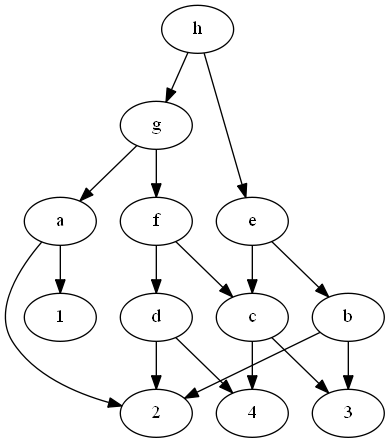# Tag Archives: PO5

## Code Generation With Unlimited Registers

The reason I found the easier reduction for the previous problem is because it was in the paper that discussed this similar problem.

The problem: Code Generation With Unlimited Registers.  This is problem PO5 in the appendix

The description: Given a Directed, Acyclic graph G=(V, A), where no vertex has an out-degree larger than 2.    Just like the previous problem, the leaves of this graph (with in-degree 0) are our starting values, and the roots of the graph (with out-degree 0) are the final values we need to compute.

The operations that are allowed are:

• ri <- rj (copy a value between registers)
• ri <- ri op rj  (replace a value in ri with the combination of ri and rj.  This is how we “compute” nodes in the graph with 2 children)

(The problem definition in G&J makes a big deal about labeling the (at most) 2 edges leaving a vertex v as “Left” and “Right”, and so the ri comes from the “Left” side, and the rj comes from the “Right” side.  But G&J also say (as a “private communication”) that this problem remains NP-Complete if we allow commutative instructions- in other words, ri <- rj op ri is also allowed.  So I don’t think it matters.)

Can we compute the root vertices of G in K or fewer instructions, if we allow any number of values to be stored in registers?

Example: Here’s the complicated graph from last time:We can start by loading the 1,2,3, and 4 into registers 1-4.  Then we can do:

• R1 <- R1 op R2 (compute a)
• R2 <- R2 op R4 (compute d)
• R3 <- R3 op R4 (compute c)
• R2 <- R2 op R3 (compute f)
• R1 <- R1 op R2 (compute g)
• R5 <- 2 (another copy)
• R6 <- 3 (another copy)
• R5 <- R5 op R6 (computing b)
• R3 <- R3 op  R5 (computing e)
• R1 <- R1 op R3 (computing h)

The reason why this is hard, even with as many registers as we want, is because the operations are “accumulator” operations- they always put the result of the operation back into one of the operand registers, killing the old value.  So if we want to use that value more than once, we either need to reload it or be very careful about the order in which we compute vertices.

If we allowed 3-register operations like rk = ri op rj, then this is easily solvable by computing values “up the graph” in basically any bottom-up order you want, putting each vertex’s value into a new register.

Reduction: This is in the paper by Aho, Johnson, and Ullman that we used last time also talks about this variant of the problem.  They say that it’s basically the same situation, you just need to load the lead values into registers right away, and you get a situation that is “similar to” the one-register machine.  I think that the basic structure of the chains we are building still holds in this new situation.

Difficulty The one-register problem is a 6 or a 7, this is probably a 5 or 6 once you have that.  If you are going to make the students walk through the whole reduction step by step in this new situation, it might be a bit harder.